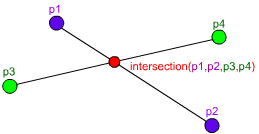## Archive for April, 2009

### Line-Line Intersection in AS3

Tuesday, April 28th, 2009I found a bug in my old Line-Line Intersection in C++ post, and after I fixed it I thought it would be a good idea to port it to AS3:

```function intersection(p1:Point, p2:Point, p3:Point, p4:Point):Point {
var x1:Number = p1.x, x2:Number = p2.x, x3:Number = p3.x, x4:Number = p4.x;
var y1:Number = p1.y, y2:Number = p2.y, y3:Number = p3.y, y4:Number = p4.y;
var z1:Number= (x1 -x2), z2:Number = (x3 - x4), z3:Number = (y1 - y2), z4:Number = (y3 - y4);
var d:Number = z1 * z4 - z3 * z2;

// If d is zero, there is no intersection
if (d == 0) return null;

// Get the x and y
var pre:Number = (x1*y2 - y1*x2), post:Number = (x3*y4 - y3*x4);
var x:Number = ( pre * z2 - z1 * post ) / d;
var y:Number = ( pre * z4 - z3 * post ) / d;

// Check if the x and y coordinates are within both lines
if ( x < Math.min(x1, x2) || x > Math.max(x1, x2) ||
x < Math.min(x3, x4) || x > Math.max(x3, x4) ) return null;
if ( y < Math.min(y1, y2) || y > Math.max(y1, y2) ||
y < Math.min(y3, y4) || y > Math.max(y3, y4) ) return null;

// Return the point of intersection
return new Point(x, y);
}```

You can try it here:

### Flash Player 10 penetration at 75.3%

Monday, April 27th, 2009

Adobe has just updated their flash penetration statistics, and flash player 10 has reached 75.3% in Europe.

Soon it will be time to make 10 the default version in my publish settings =)

### AS3 Array Shuffle

Friday, April 17th, 2009

There are a lot of ways to shuffle an array in AS3, ranging from swapping repeatedly the elements to using the sort function with a random sorter, but the most convenient way I’ve found was to simply splice a random element from the former array to the new shuffled array while the former array has elements.

DaveOnCode has a nice implementation of this method, pasted here:

```var arr2:Array = [];

while (arr.length > 0) {
arr2.push(arr.splice(Math.round(Math.random() * (arr.length - 1)), 1));
}```

Edit: Although small and convenient, this method is not the fastest way of shuffling an array, check out the comments for more info.